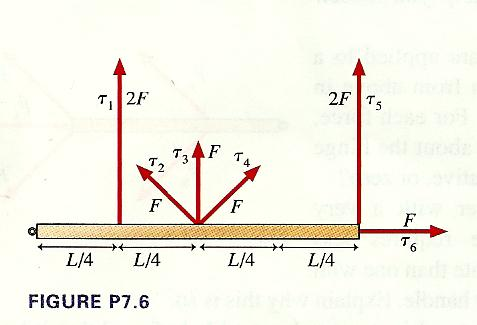# Problem: Six forces, each of magnitude either F or 2F, are applied to a door as seen from above in Figure P7.6.Rank, in order, from smallest to largest, the six torques T1 to T6 about the hinge.

###### FREE Expert Solution

Torque:

$\overline{)\begin{array}{rcl}{\mathbf{\tau }}& {\mathbf{=}}& \mathbf{r}\mathbf{×}\mathbf{F}\\ & {\mathbf{=}}& \mathbf{r}\mathbf{F}\mathbf{s}\mathbf{i}\mathbf{n}\mathbf{\theta }\end{array}}$, where r is the perpendicular distance, F is the force, and θ is the angle between the Force and the position vector, r.

𝜏1 = (L/4)(2F)sin(90°) = 0.5LF

90% (100 ratings)###### Problem Details

Six forces, each of magnitude either F or 2F, are applied to a door as seen from above in Figure P7.6.Rank, in order, from smallest to largest, the six torques T1 to T6 about the hinge.